# 4. Single Feature Representation Feedback

## 4. Single Feature Representation Feedback

A straightforward approach to multimedia retrieval is to use a single feature representation. A single feature representation is easy to extract, manage and search. In fact, text retrieval systems tend to follow this approach using a set of carefully extracted and weighted keywords along with a complex distance function. For the present discussion, we can also consider several concatenated feature representations as a single (longer) feature representation with an integrated distance function. For example, we can concatenate the color moments and wavelet texture feature representations into a larger feature and still use the Euclidean distance on the combined feature. The ImageRover  system, among others, follows this approach.

There are two main query reformulation approaches for single feature representations: single-point and multi-point. We discuss both of them in this section.

### 4.1 Single-Point Approaches

A simple query model is to use an instance of a single feature representation fi,j as a query point p in a multidimensional space, together with the corresponding set of distance functions Dij for the feature representation. One distance function dijk Dij is the "default" used by the retrieval system in the initial query. We denote the ith value (dimension) of p by p[i]. The query model is thus formed by a multidimensional point p and a distance function dijk: <p, dijk>.

Based on this model, we discuss three relevance feedback-based query formulation approaches: query point movement, affecting the shape of the distance function, and selection of the distance function itself.

#### 4.1.1 Query Point Movement

The most straightforward technique to improve a query is to change the query point itself. Selecting a different query point mimics the way a human user would go about reformulating a query herself by tinkering with the query value without modifying parameters of the distance function itself.

The objective of the query point movement approach is to construct a new query point that is "close" to relevant results, and "far" from non-relevant results. Figure 21.2 shows how this approach works for our hypothetical two-dimensional feature representation using a Euclidean distance function. The original and new query points are shown with a set of concentric circles representing iso-distance curves, that is, any point on a curve has the same distance from the query point. The query point moves to an area that is close to relevant results and away from non-relevant results.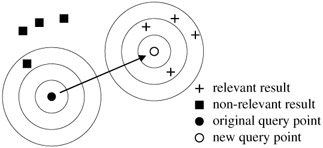Figure 21.2: Query Point Movemet

Ideally we would know, for the entire database, those results (points) that are deemed relevant, those that are deemed non-relevant, and those deemed neutral (or don't care). Under these assumptions, it can be shown that the optimal query is formed by the centroid among the relevant points minus the centroid among the remaining points. Let Srel = ai|rfi > 0 be the set of relevant points and let N be the number of points in the database, then the optimal query point popt is given by: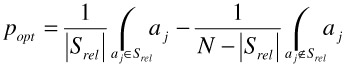In a realistic setting, however, the set of relevant points are not known a priori and must be estimated. A natural way to estimate the relevant and remaining neutral and non-relevant sets of results is to use the relevance information provided by the user.

The best-known approach to achieve query point movement is based on a formula initially developed by Rocchio  in the context of textual Information Retrieval. Let Srel be as defined above, and Snon-rel = ai|rfi < 0 be the set of points the user explicitly marked as non-relevant. The new query point is an incremental change over the original query point, which is moved towards the relevant points and away from the non-relevant points: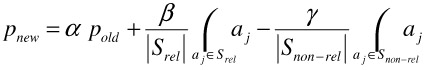The speed at which the old query point is moved towards relevant points and away from non-relevant ones is determined by the parameters α, β, and γ, where α+β+γ=1. The purpose of retaining part of the original query point is to avoid "overshooting" and to preserve some part of the user supplied sketch in the hopes it contributes important information to guide the query. In a high dimensional space it is usually difficult to determine in which direction to "go away" from non-relevant points. Although in this formula the old and relevant query points dictate this direction, it is in general advisable to make the parameter γ smaller than β to reduce any unwanted behaviour such as overshooting.

A further enhancement to this formula is to capture multiple levels of relevance by incorporating the user supplied relevance levels rfj: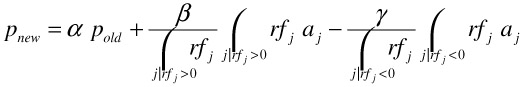As before, α, β, and γ (α+β+γ=1) control the aggressiveness with which feedback is incorporated.

The main advantages of this approach are its simplicity and generally good results. It is simple and intuitive to understand and closely mimics what a human user would do to improve a result, that is, restating her query with a different query value or sketch.

#### 4.1.2 Reshaping Distance Functions

While we used a standard Euclidean distance function throughout our discussion of the query point movement technique, there are many ways in which its shape can be influenced. Indeed, there is no restriction on the kind of distance function we can use, and its "shape" can be distorted in any arbitrary way that makes sense for that function.

The techniques we will discuss in this section focus on general LP metrics, of which Euclidian distance is only one instance. LP metrics compute the distance between two points and are of the form: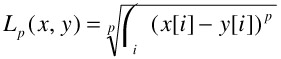The well-known Euclidean distance is thus the L2 metric, while the Manhattan distance is the L1 metric.

One approach to changing the shape of the distance function is to provide a weight for each dimension in the Lp metric. The interpretation of this is to give more importance to certain elements of the feature representation, for example, the percentage of green pixels in an image may be more important to a user than the percentage of red pixels in the image. The Lp metric becomes: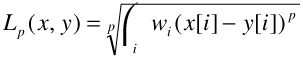where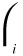, that is, all the weights add up to 1. Figure 21.3 shows how a standard Euclidean distance function changes when a weight is given for each dimension. Note that the distance function is "stretched" only along the coordinate axis.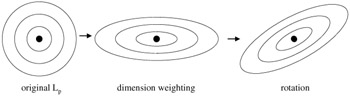Figure 21.3: Distance Function Shape

To derive a new query for the weighted LP technique, we must change the weights wi to new values that better capture the user's information need. To do this, the MARS system  suggests choosing weights wi proportional to the inverse of the standard deviation among the relevant values of dimension i, while Mindreader  suggests that using weights wi proportional to the inverse of the variance among the relevant values of dimension i provides slightly better results. The intuition behind using standard deviation or variance is that a large variation among the values of good results in a dimension means that dimension poorly captures the user's information need; conversely a small variation indicates that the dimension is important to the user's information need and should carry a higher weight.

We derive a new distance function shape through the following steps. First we estimate the new weights: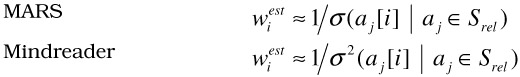Next, we normalize these weights to ensure they add up to 1 and are compatible with the original weights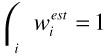. The final step is to combine the estimated weights with the original weights:As for Rocchio's formula in the previous section, parameters α and β (α+β=1) control the speed or aggressiveness of the relevance feedback, that is, how much of the original query weight is preserved for the new iteration.

While, as we pointed out earlier, giving a weight to each dimension "stretches" the distance function aligned with the coordinate axis, changes to the distance function can be further generalized by "rotating" the already stretched space as proposed by Mindreader . Figure 21.3 shows the effect of such a rotation in our hypothetical two-dimensional feature representation. The distance function must change from merely weighting individual dimensions to a more general form:

d (x,y) = (x-y)TM(x-y)

where M is a symmetric square matrix that defines a generalized ellipsoid distance and has a dimensionality equal to that of the feature representation. This rotated distance function is also known as Mahalanobis distance. We can also write this distance function as: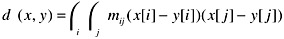where the weights mij are the coefficients of the matrix M.

To derive a new query for this distance function we must change the matrix M such that:And the determinant of M is 1 (det(M)=1). Mindreader  solves this with Lagrange multipliers and constructs an intermediate weighted covariance matrix C=[cjk]:If C-1 exists, then the M is given by:

M = (det(C))1/λC-1

where λ denotes the dimensionality of the feature representation.

To compare the dimension weighting and rotation approaches, we note that the rotation approach can capture the case where the region formed by relevant points is diagonally aligned with the coordinate axis. The dimension weighting approach is a special case of the more general rotation approach. A trivial disadvantage of the rotation model as presented in  is that it only supports a stretched and rotated version of the L2 metric; this can however easily be corrected. A more important disadvantage of the rotation approach is that it needs at least as many points to be marked relevant as the dimensionality of the feature representation to prevent the new matrix from being under-specified. This is not a problem if the feature representation has a low number of dimensions (e.g., two) but becomes unworkable if there are many dimensions (e.g., 64). The dimension weighting approach does not suffer from this limitation.

#### 4.1.3 Distance Function Selection

Another approach to constructing a new query besides selecting a new query point and changing the shape of the distance function is to find a new distance function. It may be entirely possible that something other than an L2 function or even an LP metric is what the user has in mind for a particular feature representation. The task of distance function selection is to select "the best" distance function dijk from the set Dij of distance functions that apply to the feature representation fij (as described in section 4.1).

To find the best matching distance function, we discuss an algorithm  that uses a ranked list as feedback, instead of individual relevance values rf. The user poses a query and is interested in the top m results that match her query. The objective of the retrieval system is to return to the user a ranked list <a1, a2, , am> based on the best distance function dijk. To this end, the retrieval system performs the following steps:

1. For each distance function dijkDij, the retrieval system computes an answer ranked list:

lk = <a1k,a2k,,aτmk>

where τ is a small positive integer greater than 1. That is, for each distance function it computes an intermediate answer ranked list lk larger than the user requested (typically τ=2).

2. Define a rank of operator:

 rank(as, lk) = s (rank of as in lk) if as ∈ lk rank(as, lk) = τ m+1 if as ∉ lk

The rank operator returns the position of the point as in the list lk. If as is not found in the list, we set its rank to one beyond the end of the list.

3. For each point in the lk lists, compute an overall rank rankAll(a) by combining the ranks for that point in all the lk lists. There are at most m* | Dij | different points to consider, although generally there will be many points that appear in multiple lists. We compute rankAll(a) as follows: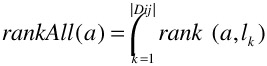4. Construct a new combined list l with the top m points based on rankAll:

l = <a1,a2,,am>

This list is presented to the user as the query result.

5. The user returns a new feedback ranked list rlf of rm points that is ranked on her perceived information need. This list contains a subset of the answer list l and is arbitrarily ordered:

rlf = <ar1,ar2,,arr>

We denote by ar points on which the user gave relevance feedback.

6. The user-supplied feedback ranked list rlf is compared to each answer list lk corresponding to a distance function in order to determine how closely they mimic the user's preferences, that is, the overall distance between their rank differences: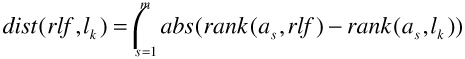7. Choose k such that the distance function dijk minimizes the overall rank differences between the user supplied rlf list and the result list lk: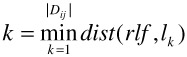In  the authors point out that this process is typically done only once. When the best distance function has been found, it remains in use during later iterations. Nothing, however, prevents an implementation from repeating this process for each relevance feedback iteration.

### 4.2 Multi-Point Approaches

In a similarity based retrieval paradigm of multimedia objects, as opposed to an exact search paradigm, it makes sense to ask for objects similar to more than one example. The user intuitively says that there is something common among the examples or sketches she supplied and wants the retrieval system to find the best results based on all the examples.

Therefore, an alternative to using a single query point p and relying on the distance function alone to "shape" the results is to use multiple query points and aggregate their individual distances to data points into an overall distance. In the single point approach, the distance function alone is responsible for inducing a "shape" in the feature space, which can be visualized with iso-distance curves. The query point merely indicates the position where this "shape" is overlaid on the feature space.

By using multiple query points and an aggregate distance function, the amount and location of query points also influences the "shape" of the query in the feature space. Using multiple query points provides enormous flexibility in the query. For example, it can overcome the limitations of the dimension weighting approach regarding diagonal queries making rotation unnecessary, and can even make dimension weighting unnecessary. Figure 21.4 shows an example of using multiple query points.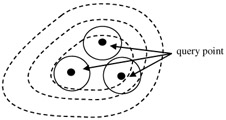Figure 21.4: Multi-point Query

To support multiple query points, we extend the query model from section 4.1 to include multiple points and a distance aggregation function. We denote the n query points by p1, p2, , pn, and the aggregate distance function for feature representation fij by Aij. The overall query model thus becomes: < (p1, p2, , pn), dijk, Aij>.

We will discuss two approaches to multi-point queries: one technique is based on the Query Expansion approach from the MARS  system, and the other one is known as FALCON . While both techniques allow the distance function dijk to be modified as discussed in section 4.1, changes to dijk are strictly independent of the present discussion and we will therefore restrict ourselves to a simple L2 metric for dijk.

#### 4.2.1 Multi-Point Query Expansion

The first approach proposes an aggregation function based on a weighted summation of the distances to the query points. Let there be a weight wt for each query point pt with 1 t n, 0 wt 1, and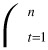, that is, there is a weight between 0 and 1 for each query point, and all weights add up to 1. The distance aggregation function Aij(x) for computing the distance of a feature representation value (i.e., of an object) x to the query is: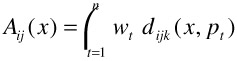Initially, all the weights are initialised to wt = 1/n , that is, they are all equal. To compute a new query using relevance feedback, two things change: (1) the set of query points pt, and (2) the weights wt that correspond to each query point pt. We discuss changing the query points next and defer a discussion of weight updating techniques to section 5, which discusses the techniques that apply here in the context of multiple features.

We present two different heuristic techniques to change the set of query points: near and distant expansion.

Near expansion: Relevant points (objects) will be added to the query if they are near objects that the user marked relevant. The rationale is that those objects are good candidates for inclusion since they are similar to other relevant objects, and thus represent other relevant objects. After the user marks all relevant objects, the system computes the similarity between all pairs of relevant and query points in order to determine the right candidates to add. For example, suppose that an initial query contains two sample points p1 and p2. The system returns a ranked list of objects <a1, a2, am>, since p1 and p2 were in the query, a1=p1 and a2=p2. The user marks a1, a2, and a4 as relevant and a3 as very relevant. Next, the system creates a distance table of relevant objects:

Point

rf

a1

a2

a3

a4

a1

1 (relevant)

0

.4

.1

.5

a2

1 (relevant)

.4

0

.4

.4

a3

2 (very relevant)

.05

.2

0

.2

a4

1 (relevant)

.5

.4

.4

0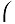.95

1

.9

1.1

The value in column r row s in the table is computed as dijk(ar,as)/rfs. Objects with a low distance are added to the query and the objects with a high distance are dropped from the query. In this example, a3 is added to the query since it has the lowest distance among points not in the query, and a2 is dropped from the query since it has the highest distance among the query points.

Distant expansion: Relevant points (objects) that are more distant to other relevant objects will be added to the query. The rationale is that if an object is relevant while different from other relevant objects, it may have some interesting characteristics that have not been captured so far. Adding it to the query will give the user an opportunity to include new information not included at the start of the query process. If the point is not useful, it will be eliminated in the next relevance feedback iteration. This approach is implemented as above, except that instead of adding objects with the lowest distance values, those with high distance values are added.

In the near and distant expansion approaches, the number of objects added during an iteration is limited to a constant number of objects (e.g., four objects) to reduce the computation cost impact.

#### 4.2.2 FALCON

The FALCON  approach to multipoint queries was specifically aimed at handling disjoint queries. Its aggregate distance function for a data point x with respect to the query is defined as: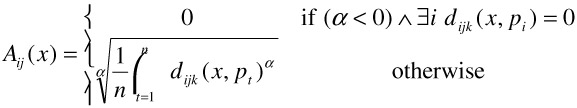This aggregate distance function is sensitive to the value of the parameter a and the location of the query points pi. When α is 1, it behaves very much like the weighted summation approach discussed above. It is when α is negative that it captures with ease disjoint queries; typical values for a are between -2 and -5. Computing a new query under this approach is as simple as adding all the results (data points) that the user marked as relevant to the query points. That is, the new query becomes <(q1, q2, , qn ai| rfi>0), dijk, Aij>. Computing a new query is therefore extremely easy to do, but comes at the cost of increased computation since potentially many query points need to be explored and evaluated. Nevertheless, FALCON is able to easily learn complex queries in the feature space, even "ring" shaped queries.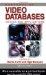Handbook of Video Databases: Design and Applications (Internet and Communications)
ISBN: 084937006X
EAN: 2147483647
Year: 2003
Pages: 393